## Friday, 8 August 2014

### CHAPTER 2 - Addition of Vectors

You will be able understand the discussion that follows very clearly only if you try to visualise everything physically. Everything about vectors will then automatically fall in place in your mind.
(A) ADDITION OF VECTORS : TRIANGLE / PARALLELOGRAM LAW
Most of you will already be very familiar with how to add vectors, from your study of physics.
Consider two vectors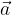$\vec a$ and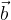$\vec b$ which we wish to add. Let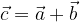$\vec c = \vec a + \vec b$
Thus,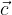$\vec c$ should have the same effect as$\vec a$ and$\vec b$ combined. To find the combined effect of$\vec a$ and$\vec b$, we place the initial point of$\vec b$ on the end-point of$\vec a$(or vice-versa):A person who starts at point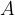$A$ and walks first along$\vec a$ and then along$\vec b$ will reach the point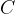$C$. Thus, the combined effect of$\vec a$ and$\vec b$ is to take the person from$A$ to$C$, i.e,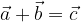$\vec a + \vec b = \vec c$ should be the vector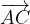$\overrightarrow {AC}$:In general, we see that to add two vectors, say$\vec a$ and$\vec b$, we place the initial point of one of them, say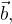$\vec b,$ at the end-point of the other, i.e.$\vec a$, . The vector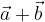$\vec a + \vec b$ is then the vector joining the tip of$\vec a$ to the end-point of$\vec b$. This is the triangle law of vector addition.$\vec a$ and$\vec b$ can equivalently be added using the parallelogram law; we make the two vectors co-initial and complete the parallelogram with these two vectors as its sides:The vector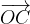$\overrightarrow {OC}$ then gives us the sum of$\vec a$ and$\vec b$.Note that the triangle and the parallelogram law are entirely equivalent; they are two slightly different forms of the same fundamental principle.
We note the following straightforward facts about addition.
(a) Existence of identity
For any vector$\vec a$,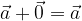$\vec a + \vec 0 = \vec a$
so that$\vec 0$ vector is the additive identity.
(b) Existence of inverse:
For any vector$\vec a$,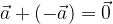$\vec a + \left( { - \vec a} \right) = \vec 0$
and thus an additive inverse exists for every vector.
(c) Commutativity:
Addition is commutative; for any two arbitrary vectors$\vec a$ and$\vec b$,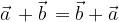$\vec a\, + \vec b\, = \vec b + \vec a$
(d) Associativity:
Addition is associative; for any three arbitrary vectors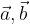$\vec a,\vec b$ and$\vec c$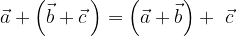$\vec a + \left( {\vec b + \vec c\,} \right) = \left( {\vec a + \vec b} \right) + \,\,\vec c$
i.e, the order of addition does not matter.
Verify this explicitly by drawing a vector diagram and using the triangle / parallelogram law of addition.